: The Digit Sum Process : Curious Properties of Reiterated : Randomness of the Digit

# 6. Another Version of Digit Sum Process

Here we are going to present a new version of dsf process. In this process we let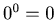, in other words, we do not use 0 when we are going to calculate dsf function with the digits.

Definition 6.1.   We define the dsf function by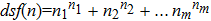, where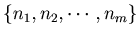is the list of the digits of an integer n such that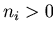.

We have chosen 1000 numbers at random, and for each of these 1000 numbers we get the list of numbers that are in the steps to the loops. We denote the odd number and the even number by 1 and 0, and we look for patterns {{0,0,0},{0,0,1},{0,1,0},{0,1,1},{1,0,0},{1,0,1},{1,1,0},{1,1,1}} in these lists of numbers. Then we have found out that the distribution of these patterns are {5931, 6444, 4821, 6548, 6893, 4742, 6679, 6670}. By this we know that the patterns {0,1,0} and {1,0,1} are relatively small compared to other patterns. Although we have not applied statistical method, the difference is almost clear. In other words this process is not random in terms of odd and even, although we have changed the definition of the process.

Example 6.1.   These are the graphs of the loops of the digit sum of Definition 6.1．The lengths of these loops are 25,52,58 respectively. We have not known that there are other loops or not.

Graph 6.1.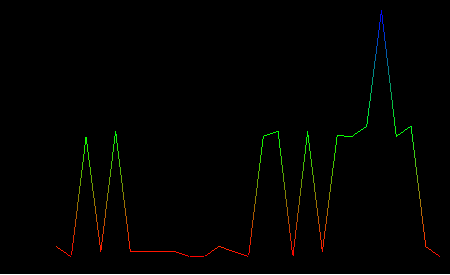Graph 6.2.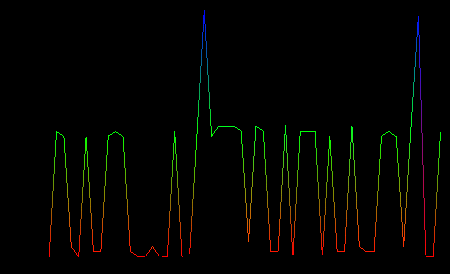Graph 6.3.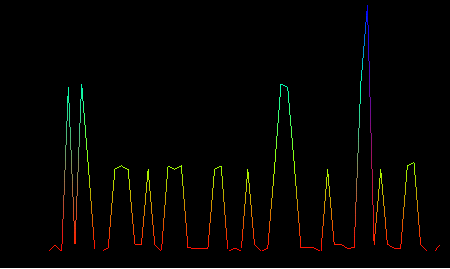: The Digit Sum Process : Curious Properties of Reiterated : Randomness of the Digit